# How to Calculate Hours Worked – Excel & Google Sheets

This tutorial will demonstrate how to calculate hours worked in Excel and Google Sheets.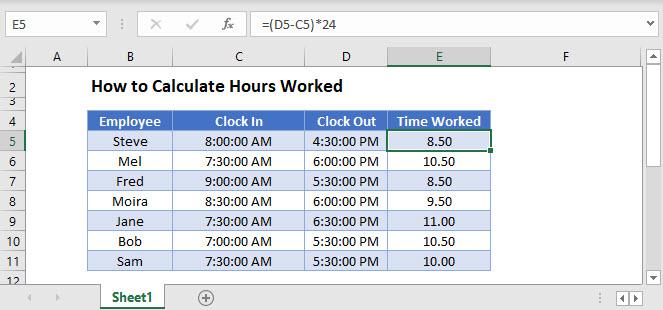## Calculate Hours with a Simple Formula

In Excel, times are stored as decimal values. Knowing this, you can simply subtract the start time (“Clock In”) from the end time (“Clock Out”) to find the time worked. (Note, we multiply by 24 to convert the decimal values into hours).

``=(D3-C3)*24``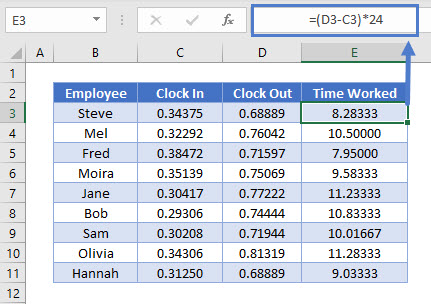In the previous, we displayed the times as decimal values to demonstrate how they are stored. We did this, by changing the formatting to “Number”):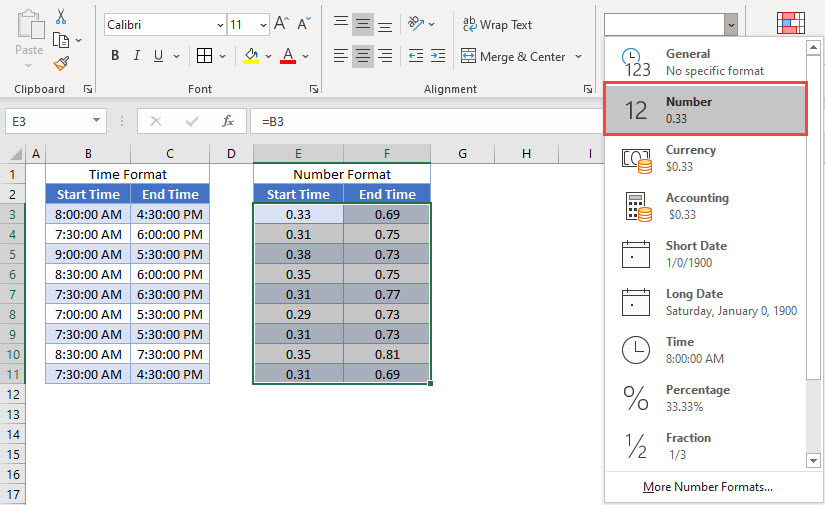## MOD Function

If the end time is before the start time (ex. night shift) the formula will return a negative number. To solve this problem, use the MOD Function.

``=MOD(D3-C3,1)*24``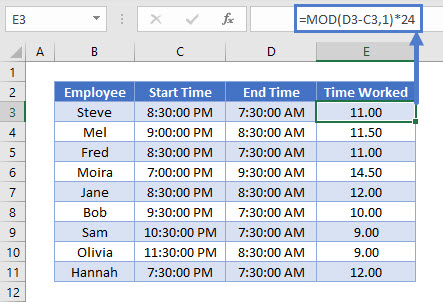As with the simple formula, we need to multiply the value calculated by the MOD Function by 24 to get the hours worked.

## Calculating Overtime

We can use the same type of formula to calculate overtime.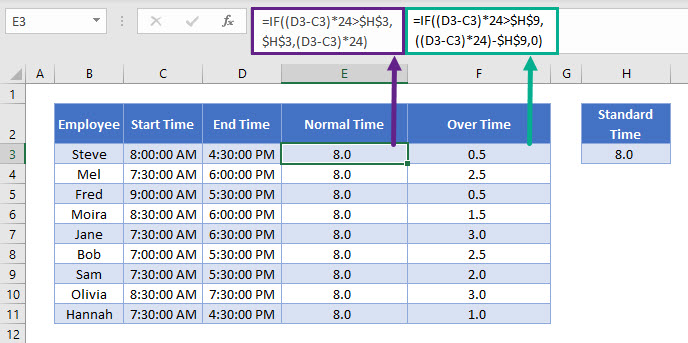In the example above, the standard time is 8 hours.

The following formula calculates the Normal time worked in the day.

``=IF((D3-C3)*24>\$H\$13,\$H\$13,(D3-C3)*24)``

If the employee has worked more than 8 hours, the formula will only return a maximum of 8 hours.

To calculate the overtime, we can get the rest of the hours worked using this formula below:

``=IF((D3-C3)*24>\$H\$13,((D3-C3)*24)-\$H\$13,0)``

## Calculate Hours Worked in a Weekly Timesheet

We can use the IF, SUM and MAX Functions to calculate the hours worked in a weekly broken down by regular time and overtime.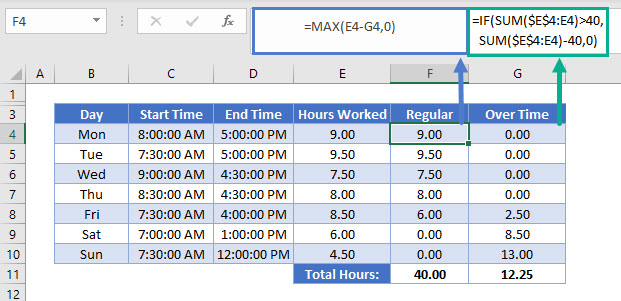### Calculate Overtime

The overtime is calculated once a person works more than 40 hours a week.

``=IF(SUM(\$E\$4:E4)>40,SUM(\$E\$4:E4)-40,0)``

The first part of the first range of the SUM Function is an absolute, while the second part is not.  As you copy this formula down to the bottom of the table, you will notice that the SUM function adds up all the Hours worked in column E.  As the SUM range is increased, so the hours worked is increased.  Once the SUM gets to more than 40 hours, Overtime hours are put into the Overtime column as an increasing total.

### Calculate Regular Hours

The regular hours are calculated based on the total hours, and the overtime worked.

``=MAX(E4-G4,0)``

We use the MAX Function so that we do not end up with Negative hours where the Employee has worked overtime as if the result returns a negative, then the MAX function will return a zero.

### Easy Excel Automation## Calculate Hours Worked in a Weekly Timesheet by Project

Taking the calculation above one step further, we can divide the hours worked by the Project that the employee worked on using the SUMIF Function

``=SUMIF(\$H\$4:\$H\$10,H4,\$F\$4:\$F\$10)``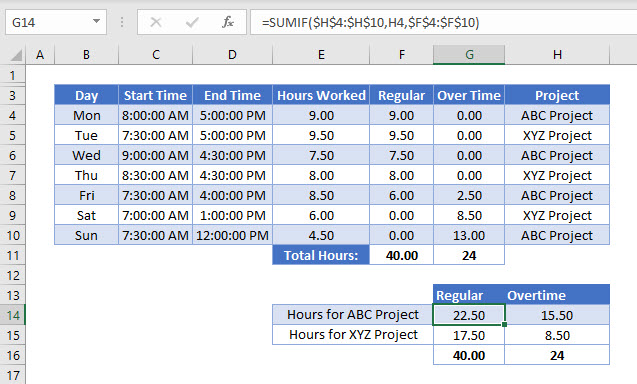The SUMIF function will sum the Regular hours in column F according to the criteria selected in column H – in the case of the above formula, it will look for the ABC Project and sum the Regular hours for that project.

## Calculating Hours Worked in Google Sheets

All the above Excel examples work the same way in Google Sheets.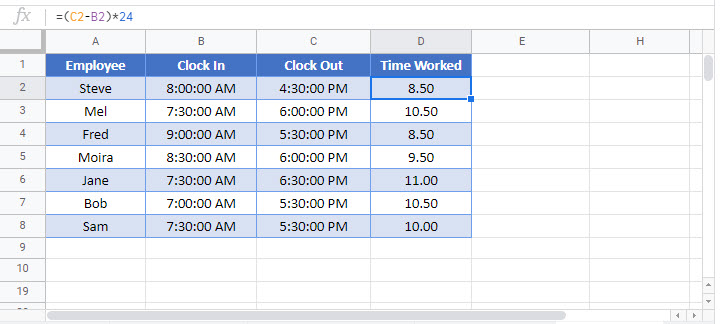### Excel Practice Worksheet

Practice Excel functions and formulas with our 100% free practice worksheets!